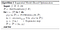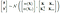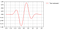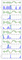# Deep-dive in Bayesian Hyper-Parameter Tuning

An intuition and implementation summary of Sequential Model-Based Optimization Algorithm for Bayesian Hyper-Parameter Tuning

# Model Hyper-Parameter vs Model Parameter

For a machine learning Model, Model Hyper-Parameters are set to a model before training and are static during training.

Model Hyper-Parameters includes

1. Model layout and Model attributes, like number of trees and max-depth of tree for Random Forest, number of layers and number of units in each layer for Neural Network, number of clusters for KNN etc
2. Training settings, like learning rate, regularization rate, batch-size etc

Model parameters, on the other hand, are dynamic evolved during the training, and are learned from training data to make model fit training data.

Model Parameters includes

1. Weights or Coefficients for Regression Model
2. Split Condition for Decision Tree
3. KNN Centroid
4. etc

# Bayesian Hyper-Parameter Tuning Conceptual Model

Bayesian Hyper-Parameter Tuning implementation is based on the below Sequential Model-Based Optimization (SMBO) algorithm.Bayesian Optimization Primer

X: is hyper-parameters space

f : is a black box function yᵢ = f (xᵢ), xᵢ is one sample from hyper-parameters space. Here f (xᵢ) can be a model loss function with model hyper-parameters xᵢ

D: is dataset {(x₁, y₁), . . . , (xₙ , yₙ)}, different hyper-parameters sample xᵢ with its corresponding model performance metric yᵢ

M: is Statistical Surrogate Model which fits dataset D

S: is Acquisition Function which uses statistical model M to select next hyper-parameters sample xᵢ which may get better model performance metric yᵢ

The ultimate purpose of SMBO is to estimate the conditional distribution p(y|x ), so based on the distribution, we can find the hyper-parameters x which has best model performance y.

# Statistical Surrogate Model (M)

In SMBO algorithm, M is a statistical model which fit sampled dataset D {(x₁, y₁), . . . , (xₙ , yₙ)}.

Practically, there are three ways to discover model M

1. Gaussian Processes (GPs)
2. Tree Parzen Estimators (TPE)
3. Random Forests

## Intuition using Gaussian Processes

Dataset D {(x₁, y₁), . . . , (xₙ , yₙ)} can be a n-dimensional space, the dimensions are X = [x₁, x₂, . . . , xₙ]

Assume y = [y₁, y₂, . . . , yₙ] follows the below multivariate Gaussian Distribution

P ( y, X) ~ N ( μ , K )

μ =[ m(x₁), . . . , m(xₙ) ], m represents the mean function

K is covariance matrix, each matrix value k( xᵢ , xⱼ ) are defined by a kernel function, and if points xᵢ and xⱼ are considered to be similar by the kernel, then yᵢ and yⱼ are expected to be similar.

If a new sample (X* , y*) added to dataset D, then the joined distribution (y , y*) is expressed as below, and it is still multivariate Gaussian DistributionFor details of the formula please reference Bayesian Optimization Primer

So we can follow the process, sampling x*, calculating y* = f (x*), then updating the join distribution (y , y*).

Once got enough sampling, the distribution will be accurate to model the relationship of x and y.

Next step, we need convert the join distribution P (y, y*, X, X*) into a conditional distribution P ( y* | y, X, X*) as below, so we can predict output y* for any X*.Bayesian Optimization Primer

Gaussian process is quite complex , the above is just a general steps for Gaussian Process under my understanding.

## Intuition using Tree Parzen Estimators (TPE)

Different from GP, TPE doesn’t directly construct a statistical model P ( y | x ), instead TPE choose to calculate distribution P (x | y) as below:

y* is a threshold value, for example we can choose a medium value of y from dataset D {(x₁, y₁), . . . , (xₙ , yₙ)}

l (x) is x distribution when y = f (x) < y*

g (x) is x distribution when y = f (x) ≥ y*

Next, TPE choose next sample X* based on the below expression (if smaller f(x) value means better model performance)

Then, calculate y* = f (X*) and append (X*, y*) to dataset D.

This is one iteration of TPE process, next TPE will repeat re-calculating l (x) and g (x), sampling X* until reaching Pre-Set number of iterations

## Intuition using Random Forests

This method is aimed to used a Gaussian Distribution y~ N( X ) to model the dataset D {(x₁, y₁), . . . , (xₙ , yₙ)}

Assume a Random Forest r(x) with B trees is fit to dataset D, then the Gaussian Distribution definition is as below:

# Acquisition Function (S)

Acquisition function is the sampling criteria by which the next sample of hyper-parameters are chosen from hyper-parameters space.

The criteria will find new samplers by exploration and exploitation in the hyper-parameters space.

Exploration means try sampling from the areas have large uncertainty (variance).

Exploitation means sample the areas we already know have good performance.

Below are two most popular acquisition function:

## Probability of Improvement (PI)

Probability of Improvement is try to find the sample which have the max-probability to improve the model loss function.

Assume in current sample dataset D {(x₁, y₁), . . . , (xₙ , yₙ)}, the minimal yᵢ is y*, then PI is P( y < y* ) and the next sample x is argmax ( P( y < y* ) )

If we use Gaussian Process to model dataset D, the PI expression is

ϕ is gaussian cumulative distribution function

The next sample is

## Expected Improvement (EI)

PI is focus on choosing sample which has max probability to improve the model loss function, but Expected Improvement is focus on how much the sampler can improve, and choose the sample which can maximize the improvement.

In Gaussian Process mode, the EI expression is

The next sample is

Base on EI expression, the next sample should be a trade-off minimize μ (x) and maximize κ(x, x)

## Example for Gaussian Process and Acquisition Function

The example will use Gaussian Process to fit the below object function f.

(scikit-optimize python library is used for Gaussian Process Regression)

`import numpy as npdef f(x):    return np.sin(5 * x) * (1 - np.tanh(x ** 2))`Object Function f

Fit function f use Gaussian Process Regression and EI Acquisition Function

`from skopt import gp_minimizeimport matplotlib.pyplot as pltfrom skopt.plots import plot_gaussian_processres = gp_minimize(f,                       [(-2.0, 2.0)],                        acq_func="EI",                  n_random_starts=8,                    random_state=1234)plt.rcParams["figure.figsize"] = (10, 30)n_samples = 6for n_iter in range(n_samples):    plt.subplot(n_samples*2, 1, 2*n_iter+1)    if n_iter == 0:        show_legend = True    else:        show_legend = False            plot_gaussian_process(res,                           n_calls=n_iter,                          objective=f,                          show_legend=False,                           show_title=False)        # Plot AC    plt.subplot(n_samples*2, 1, 2*n_iter+2)    plot_gaussian_process(res,                           n_calls=n_iter,                          show_legend=False,                           show_title=False,                          show_mu=False,                           show_acq_func=True,                          show_observations=False,                          show_next_point=True)`Gaussian Process and EI

# Example for Bayesian Hyper-Parameter Tuning

This example uses scikit-optimize python library and Gaussian Processes Surrogate Model to find the best hyper-parameters for Gradient Boosting Regressor

`import numpy as npfrom sklearn.datasets import load_bostonfrom sklearn.ensemble import GradientBoostingRegressorfrom sklearn.model_selection import cross_val_scorefrom skopt import gp_minimizefrom skopt.space import Real, Integerfrom skopt.utils import use_named_argsboston = load_boston()X, y = boston.data, boston.targetn_features = X.shape# gradient boosted trees hyper-parameter spacespace  = [Integer(1, 5, name='max_depth'),          Real(10**-5, 10**0, "log-uniform", name='learning_rate'),          Integer(1, n_features, name='max_features'),          Integer(2, 100, name='min_samples_split'),          Integer(1, 100, name='min_samples_leaf')]@use_named_args(space)def objective(**params):    reg = GradientBoostingRegressor(n_estimators=50, random_state=0)    reg.set_params(**params)    return -np.mean(        cross_val_score(            reg,             X,             y,             cv=5,             n_jobs=-1,            scoring="neg_mean_absolute_error"))res_gp = gp_minimize(objective, space, acq_func ="EI", n_calls=100, random_state=0)print("""Best parameters:- max_depth=%d- learning_rate=%.6f- max_features=%d- min_samples_split=%d- min_samples_leaf=%d""" % (res_gp.x, res_gp.x,                            res_gp.x, res_gp.x,                            res_gp.x))`

The second example uses hyperopt library and Tree Parzen Estimators (TPE) Surrogate Model

`import numpy as npimport pandas as pdfrom hyperopt.pyll.stochastic import samplefrom hyperopt import tpe, hp, fmin, STATUS_OK,Trialsfrom sklearn.datasets import load_bostonfrom sklearn.ensemble import GradientBoostingRegressorfrom sklearn.model_selection import cross_val_scoreboston = load_boston()X, y = boston.data, boston.targetn_features = X.shape# gradient boosted trees hyper-parameter spacespace = {    "max_depth": hp.choice('max_depth', range(1, 5, 1)),    "learning_rate": hp.loguniform('learning_rate',np.log(0.005),np.log(0.1)),    "max_features": hp.choice("max_features", range(1,n_features,1)),    "min_samples_split": hp.choice("min_samples_split", range(2,100,1)),    "min_samples_leaf": hp.choice("min_samples_leaf", range(1,100,1))}def hyperparameter_tuning(params):    reg = GradientBoostingRegressor(n_estimators=50, random_state=0)    reg.set_params(**params)    mae = -np.mean(        cross_val_score(            reg,             X,             y,             cv=5,             n_jobs=-1,            scoring="neg_mean_absolute_error"))    return {"loss": mae, "status": STATUS_OK}trials = Trials()best = fmin(    fn=hyperparameter_tuning,    space = space,     algo=tpe.suggest,     max_evals=100,     trials=trials)print("""Best parameters:- max_depth=%d- learning_rate=%.6f- max_features=%d- min_samples_split=%d- min_samples_leaf=%d""" % (best["max_depth"],                            best["learning_rate"],                            best["max_features"],                            best["min_samples_split"],                            best["min_samples_leaf"], ))`

# Final Summary

Based on the above demo tests, different Surrogate Model may get different Best_Parameters, it is funny. And even for same Surrogate Model, if the initial samples are different, the results may different due to local minimal trap and different scale of exploration and exploitation. So we need to tune the parameters for the algorithm to tune the model hyper-parameters. My current conclusion for Bayesian Hyper-Parameter Tuning is, it can be a reference but not a silver-bullet to handle model hyper-parameter tuning in practical project.

# REFERENCE

Data Scientist & Engineer from Sydney

## More from Summer Hu

Data Scientist & Engineer from Sydney